# Lattice Spinor Gravity Quantum gravity Quantum field theory

• Slides: 56Lattice Spinor Gravity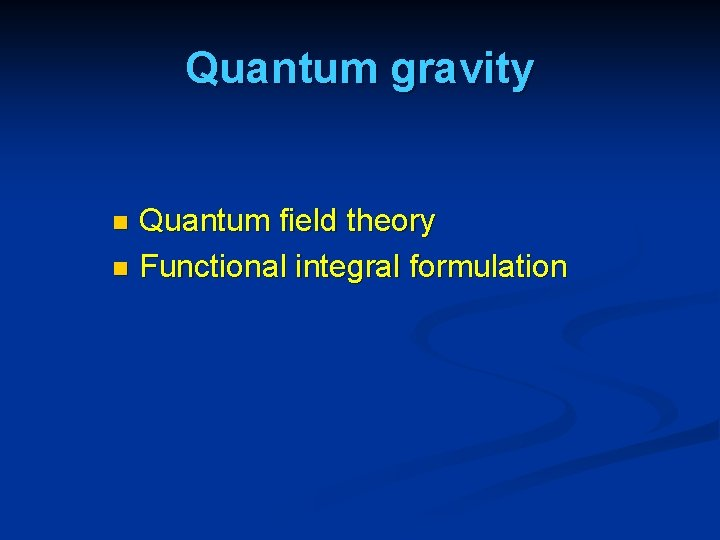Quantum gravity Quantum field theory n Functional integral formulation n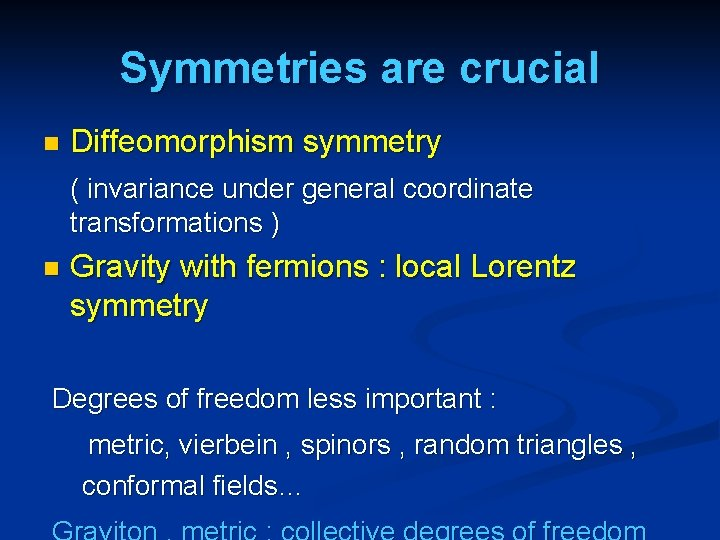Symmetries are crucial n Diffeomorphism symmetry ( invariance under general coordinate transformations ) n Gravity with fermions : local Lorentz symmetry Degrees of freedom less important : metric, vierbein , spinors , random triangles , conformal fields…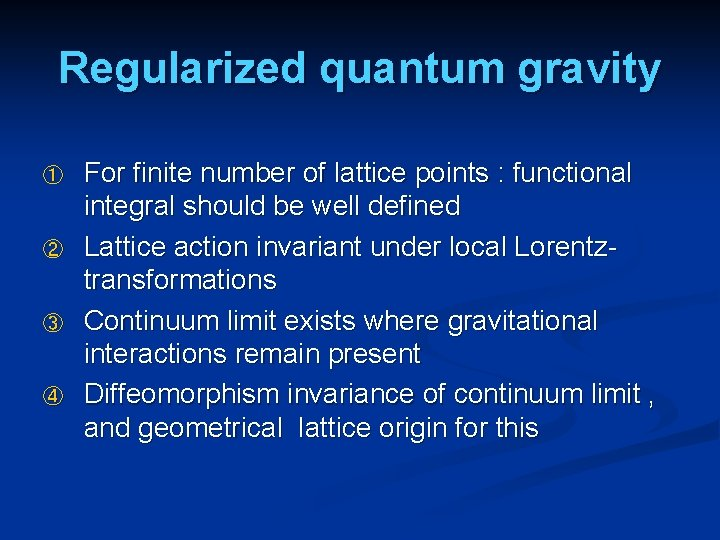Regularized quantum gravity ① ② ③ ④ For finite number of lattice points : functional integral should be well defined Lattice action invariant under local Lorentztransformations Continuum limit exists where gravitational interactions remain present Diffeomorphism invariance of continuum limit , and geometrical lattice origin for this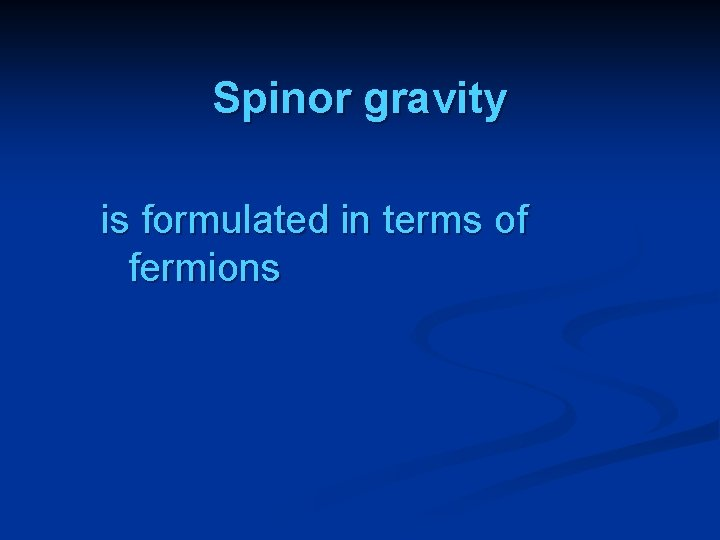Spinor gravity is formulated in terms of fermionsUnified Theory of fermions and bosons Fermions fundamental Bosons collective degrees of freedom n n n Alternative to supersymmetry Graviton, photon, gluons, W-, Z-bosons , Higgs scalar : all are collective degrees of freedom ( composite ) Composite bosons look fundamental at large distances,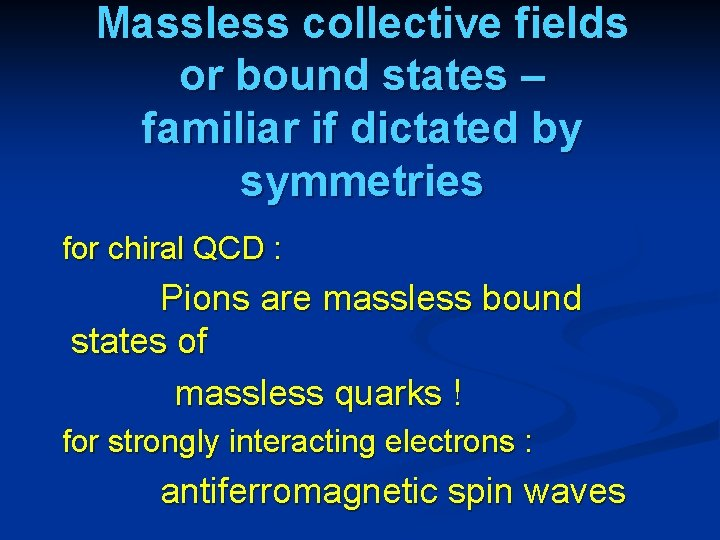Massless collective fields or bound states – familiar if dictated by symmetries for chiral QCD : Pions are massless bound states of massless quarks ! for strongly interacting electrons : antiferromagnetic spin waves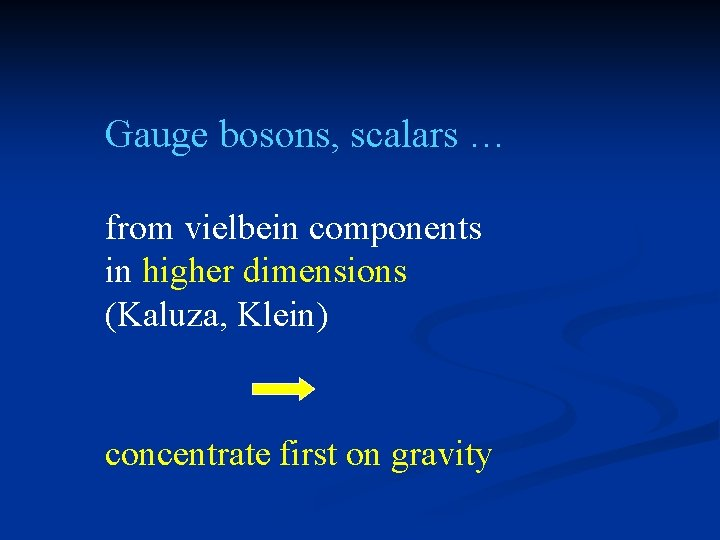Gauge bosons, scalars … from vielbein components in higher dimensions (Kaluza, Klein) concentrate first on gravity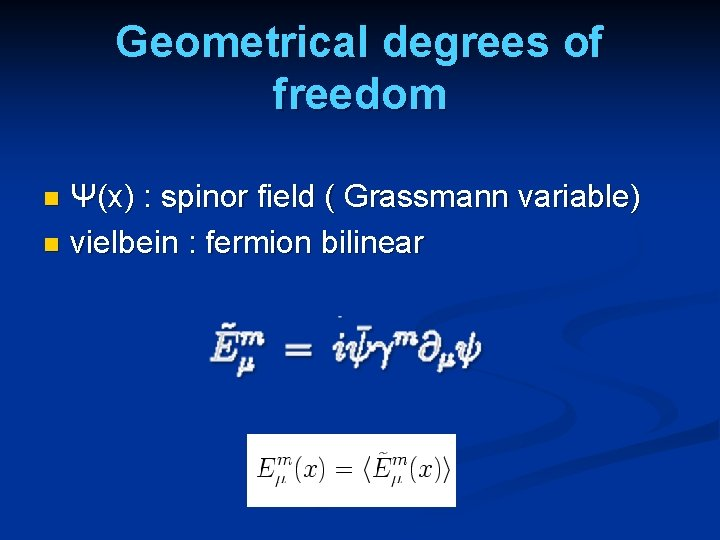Geometrical degrees of freedom Ψ(x) : spinor field ( Grassmann variable) n vielbein : fermion bilinear n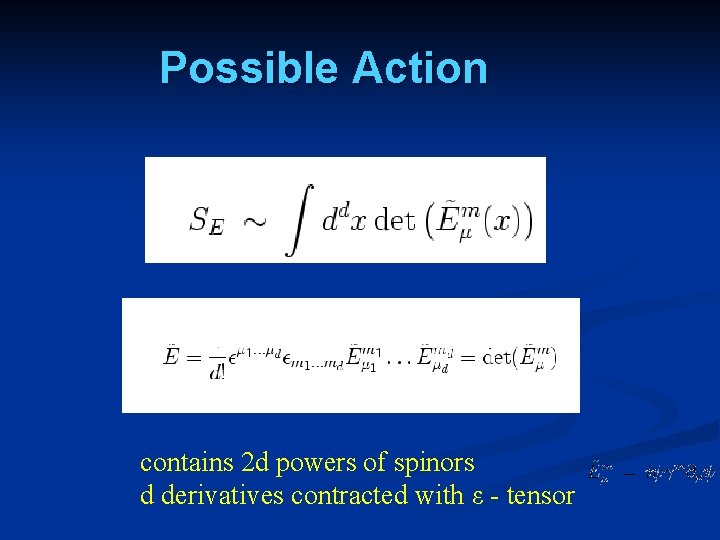Possible Action contains 2 d powers of spinors d derivatives contracted with ε - tensor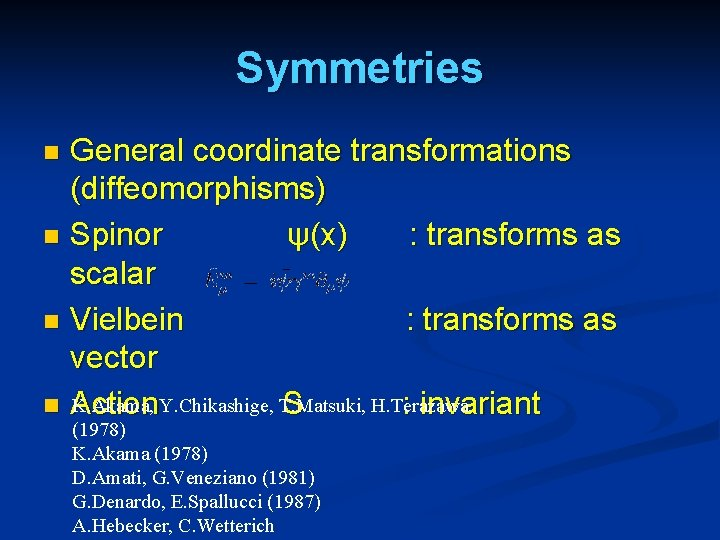Symmetries General coordinate transformations (diffeomorphisms) n Spinor ψ(x) : transforms as scalar n Vielbein : transforms as vector K. Akama, Y. Chikashige, T. Matsuki, H. Terazawa n Action S : invariant n (1978) K. Akama (1978) D. Amati, G. Veneziano (1981) G. Denardo, E. Spallucci (1987) A. Hebecker, C. Wetterich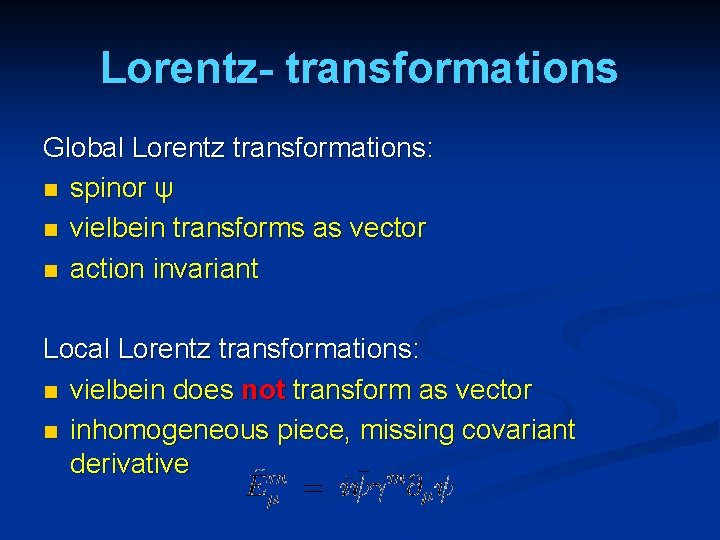Lorentz- transformations Global Lorentz transformations: n spinor ψ n vielbein transforms as vector n action invariant Local Lorentz transformations: n vielbein does not transform as vector n inhomogeneous piece, missing covariant derivativeTwo alternatives : 1) Gravity with global and not local Lorentz symmetry ? Compatible with observation ! 2) Action with local Lorentz symmetry ? Can be constructed !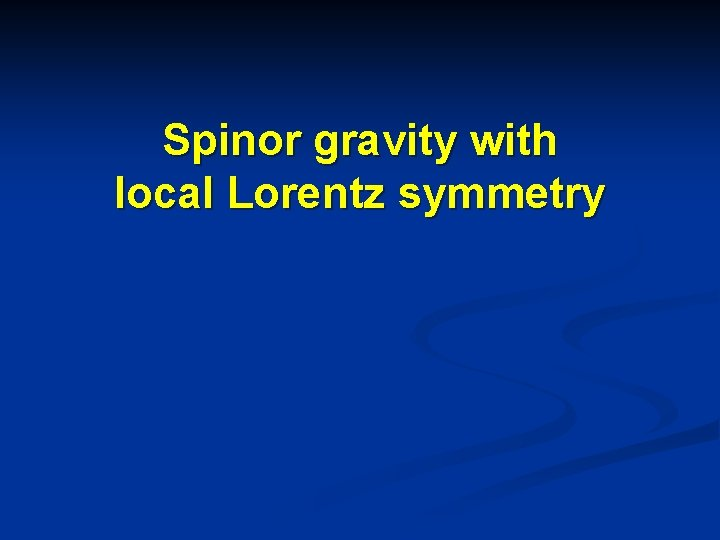Spinor gravity with local Lorentz symmetrySpinor degrees of freedom Grassmann variables n Spinor index n Two flavors n Variables at every space-time point n n Complex Grassmann variables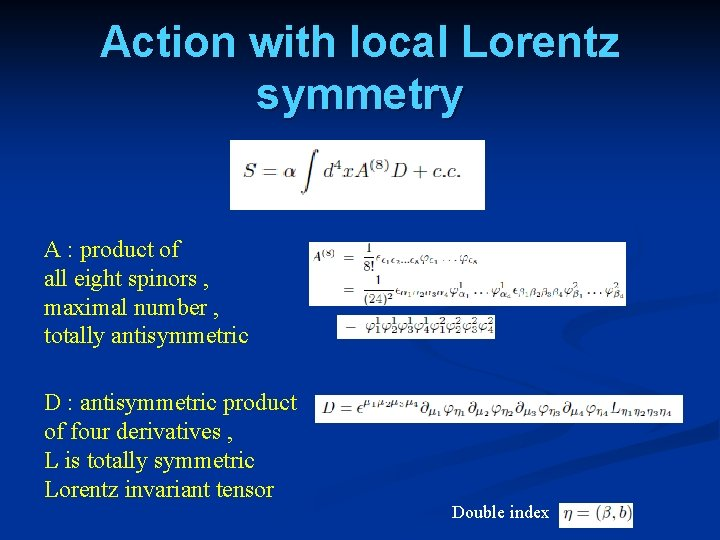Action with local Lorentz symmetry A : product of all eight spinors , maximal number , totally antisymmetric D : antisymmetric product of four derivatives , L is totally symmetric Lorentz invariant tensor Double indexSymmetric four-index invariant Symmetric invariant bilinears Lorentz invariant tensors Symmetric four-index invariant Two flavors needed in four dimensions for this construction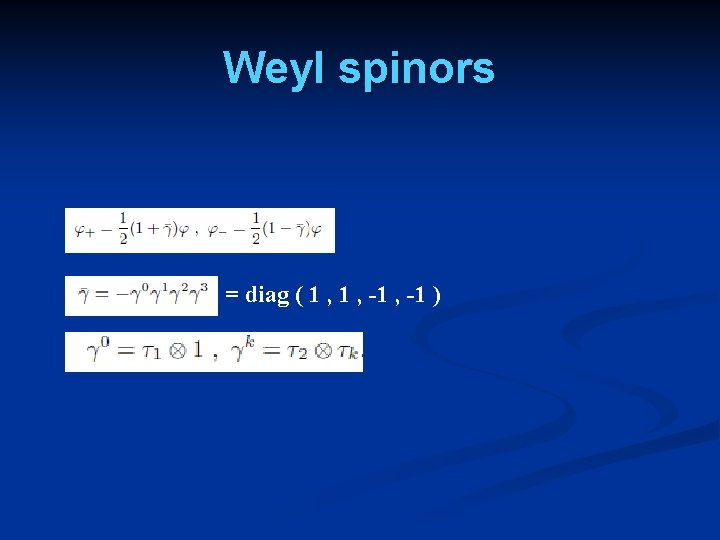Weyl spinors = diag ( 1 , -1 )Action in terms of Weyl spinors Relation to previous formulationSO(4, C) - symmetry Action invariant for arbitrary complex transformation parameters ε ! Real ε : SO (4) - transformationsSignature of time Difference in signature between space and time : only from spontaneous symmetry breaking , e. g. by expectation value of vierbein – bilinear !Minkowski - action Action describes simultaneously euclidean and Minkowski theory ! SO (1, 3) transformations :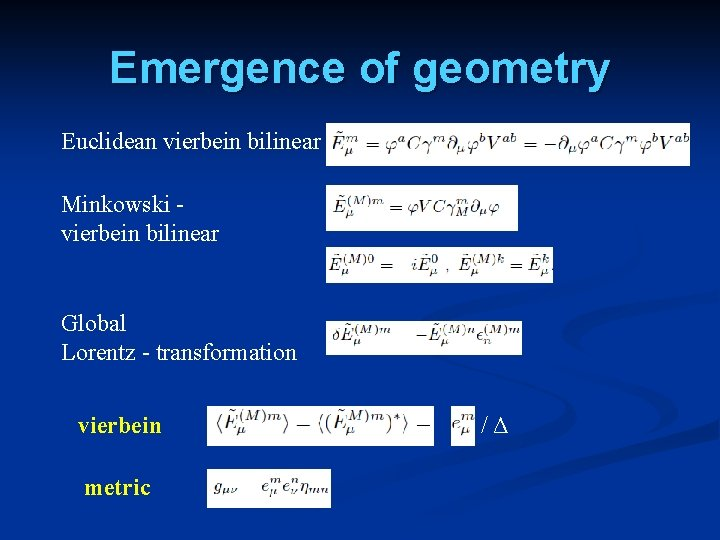Emergence of geometry Euclidean vierbein bilinear Minkowski vierbein bilinear Global Lorentz - transformation vierbein metric /Δ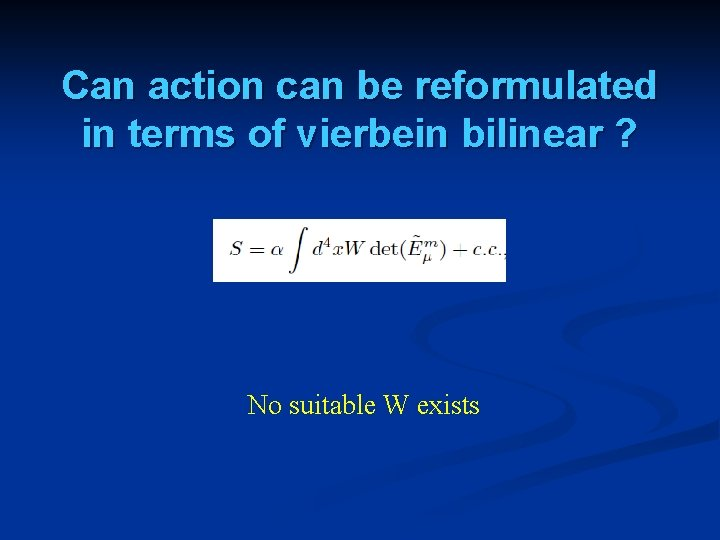Can action can be reformulated in terms of vierbein bilinear ? No suitable W exists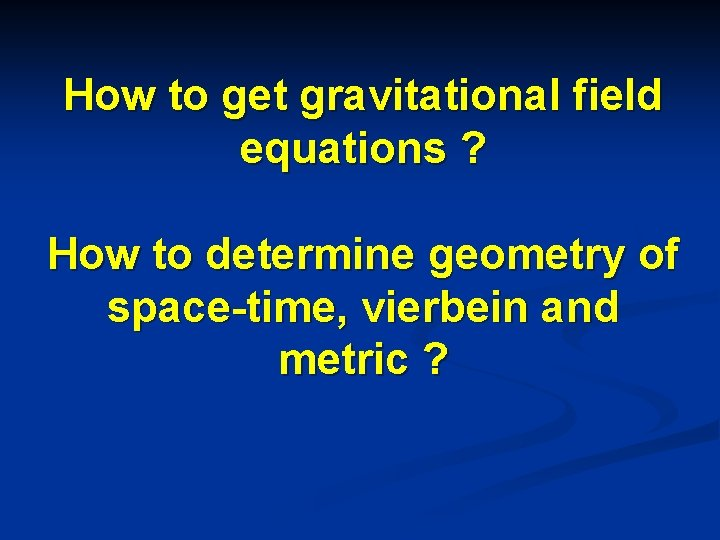How to get gravitational field equations ? How to determine geometry of space-time, vierbein and metric ?Functional integral formulation of gravity Calculability ( at least in principle) n Quantum gravity n Non-perturbative formulation n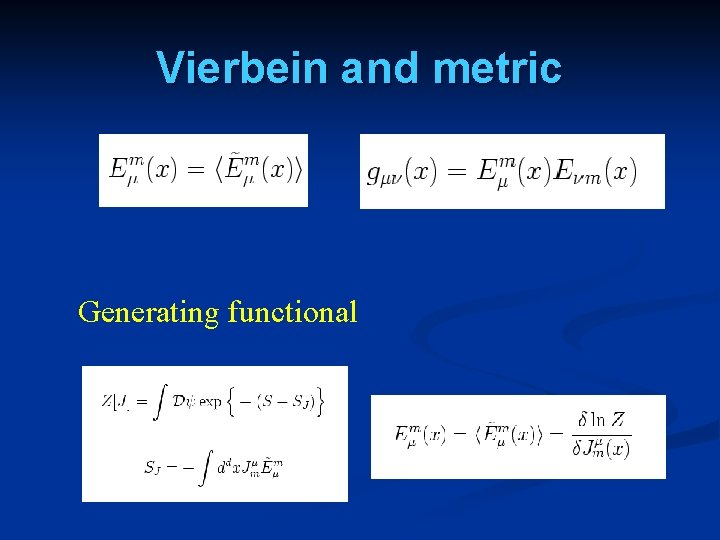Vierbein and metric Generating functional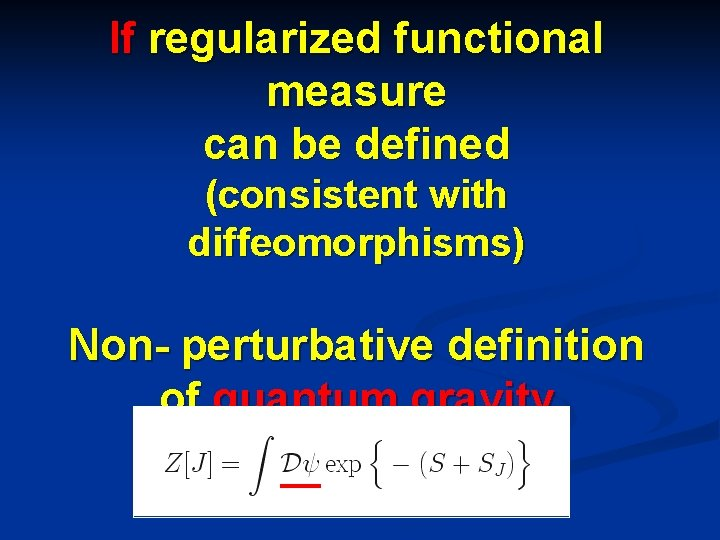If regularized functional measure can be defined (consistent with diffeomorphisms) Non- perturbative definition of quantum gravityEffective action W=ln Z Gravitational field equation for vierbein similar for metric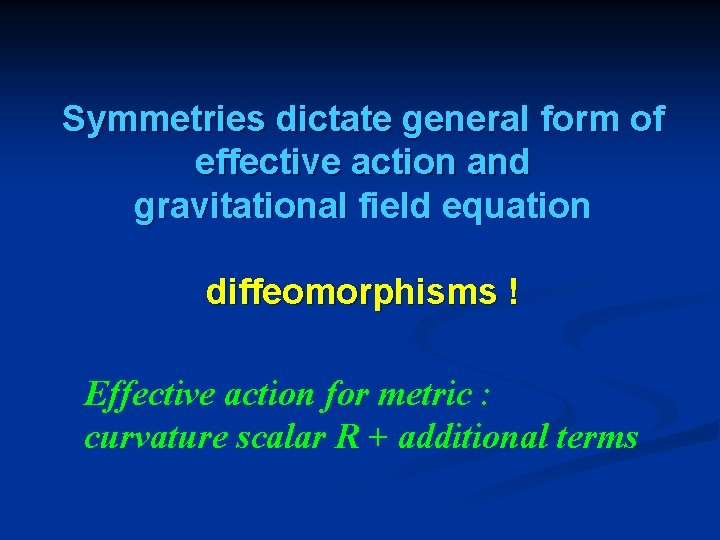Symmetries dictate general form of effective action and gravitational field equation diffeomorphisms ! Effective action for metric : curvature scalar R + additional termsLattice spinor gravity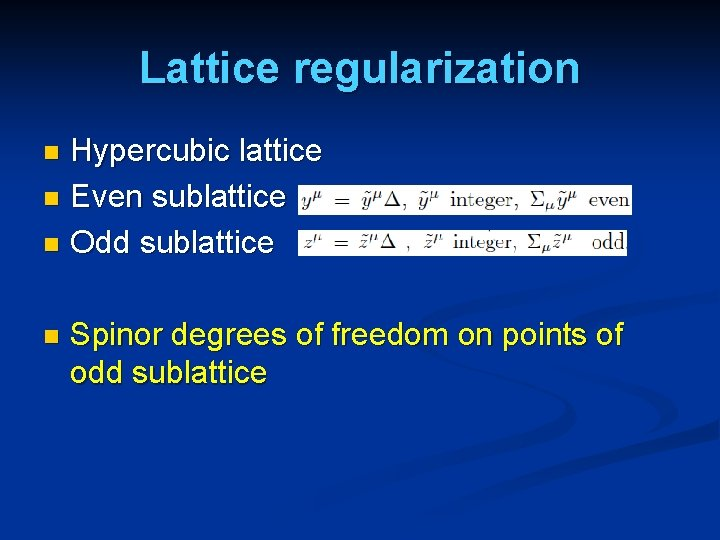Lattice regularization Hypercubic lattice n Even sublattice n Odd sublattice n n Spinor degrees of freedom on points of odd sublatticeLattice action Associate cell to each point y of even sublattice n Action: sum over cells n n For each cell : twelve spinors located at nearest neighbors of y ( on odd sublattice )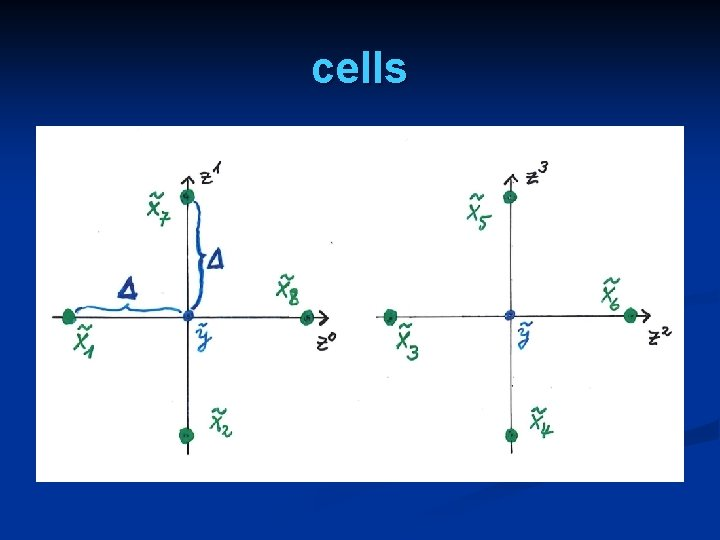cellsLocal SO (4, C ) symmetry Basic SO(4, C) invariant building blocks Lattice action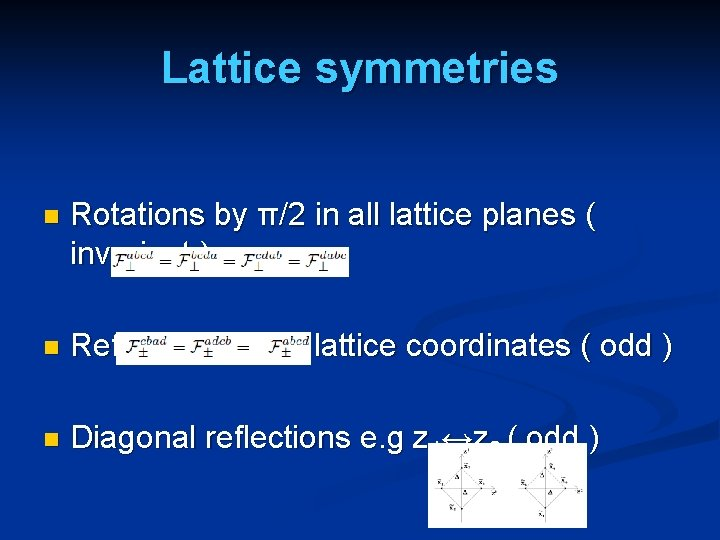Lattice symmetries n Rotations by π/2 in all lattice planes ( invariant ) n Reflections of all lattice coordinates ( odd ) n Diagonal reflections e. g z 1↔z 2 ( odd )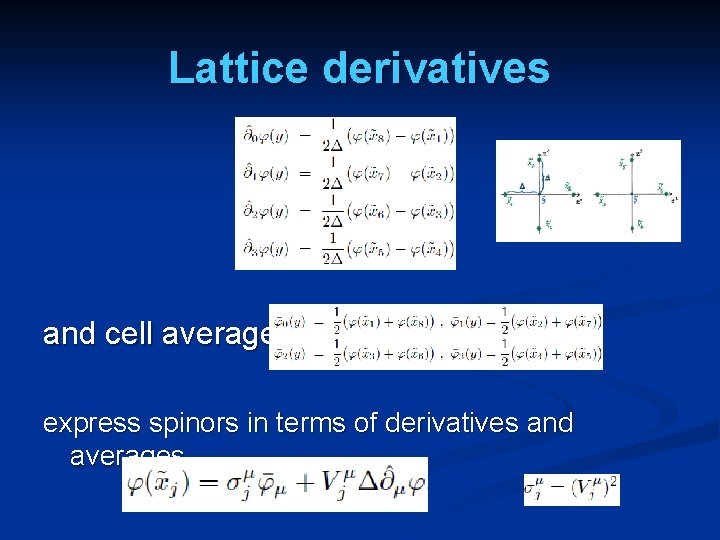Lattice derivatives and cell averages express spinors in terms of derivatives and averagesBilinears and lattice derivatives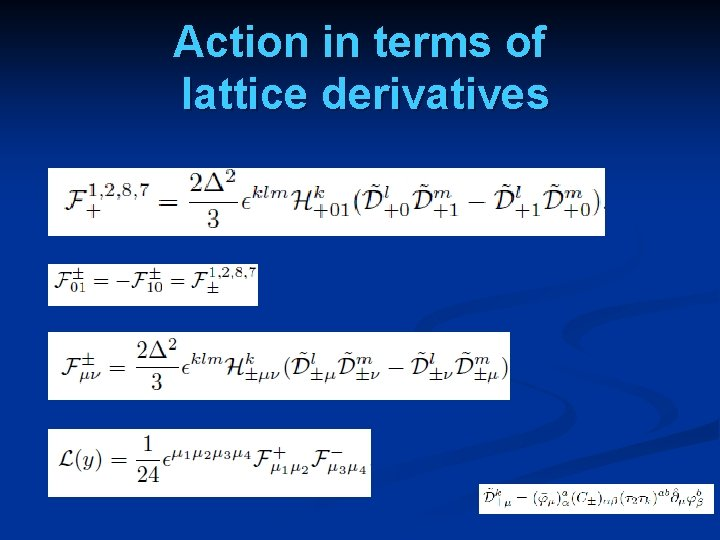Action in terms of lattice derivativesContinuum limit Lattice distance Δ drops out in continuum limit !Regularized quantum gravity n n For finite number of lattice points : functional integral should be well defined Lattice action invariant under local Lorentztransformations Continuum limit exists where gravitational interactions remain present Diffeomorphism invariance of continuum limit , and geometrical lattice origin for thisLattice diffeomorphism invariance n n Lattice equivalent of diffeomorphism symmetry in continuum Action does not depend on positioning of lattice points in manifold , once formulated in terms of lattice derivatives and average fields in cells Arbitrary instead of regular lattices Continuum limit of lattice diffeomorphism invariant action is invariant under general coordinate transformations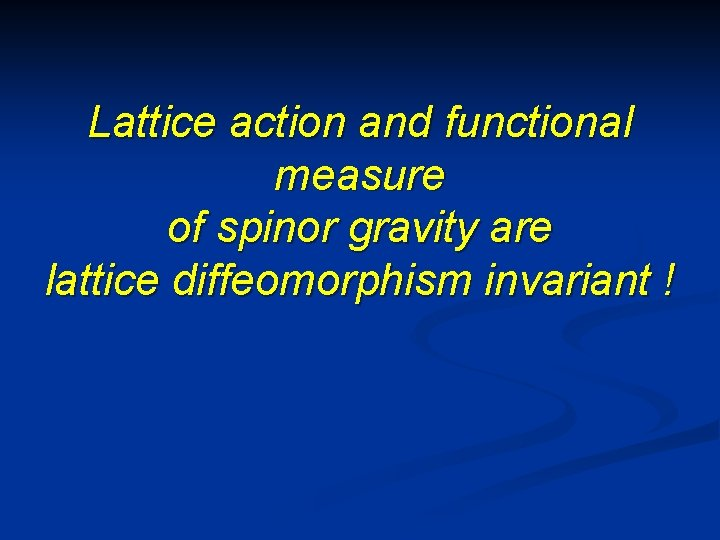Lattice action and functional measure of spinor gravity are lattice diffeomorphism invariant !Lattice action for bosons in d=2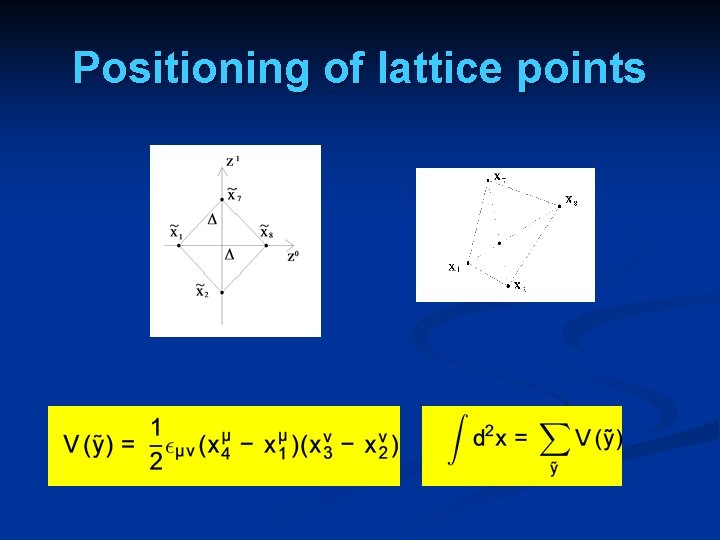Positioning of lattice points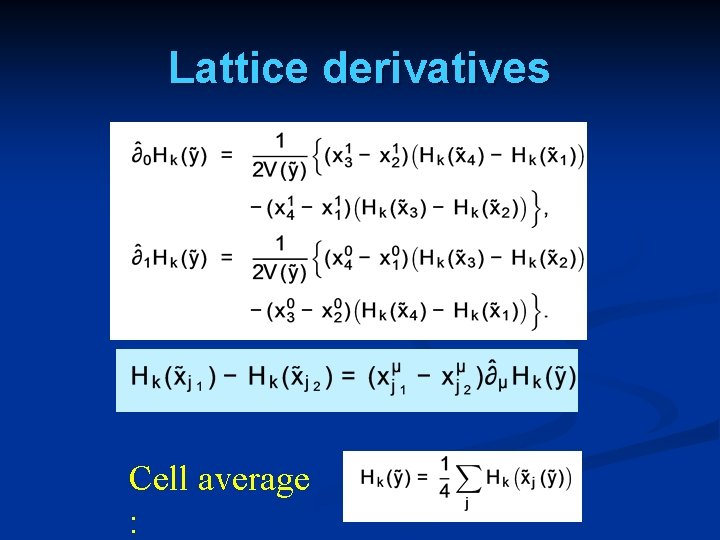Lattice derivatives Cell average :Lattice diffeomorphism invariance Continuum Limit :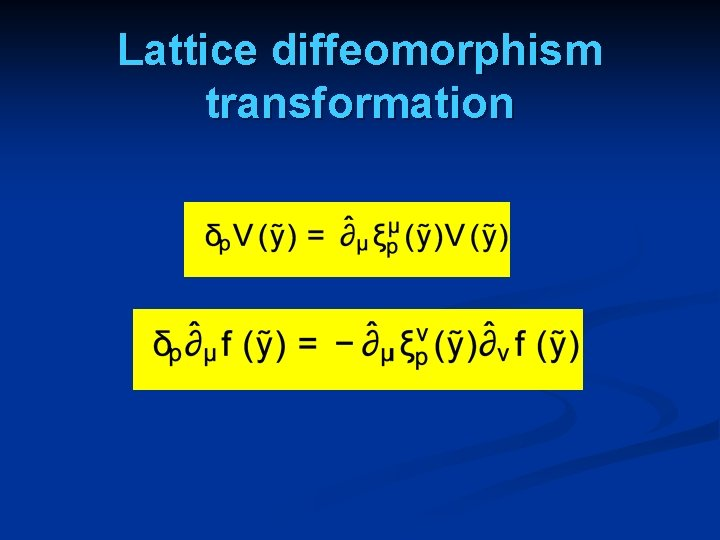Lattice diffeomorphism transformation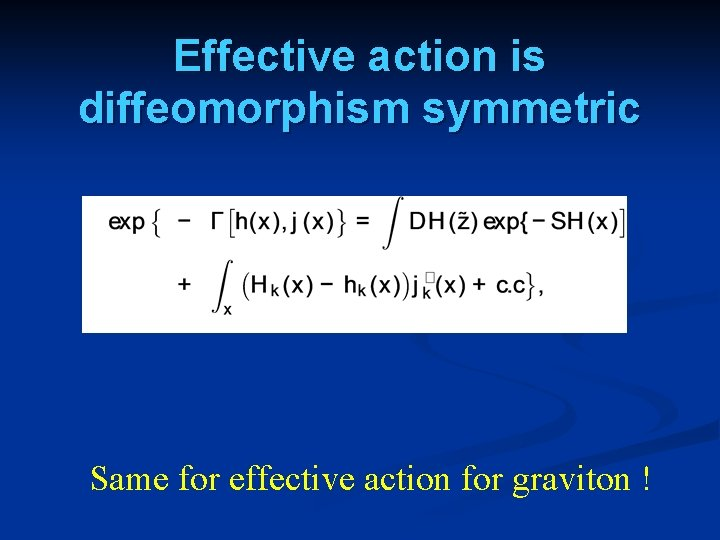Effective action is diffeomorphism symmetric Same for effective action for graviton !Lattice action and functional measure of spinor gravity are lattice diffeomorphism invariant !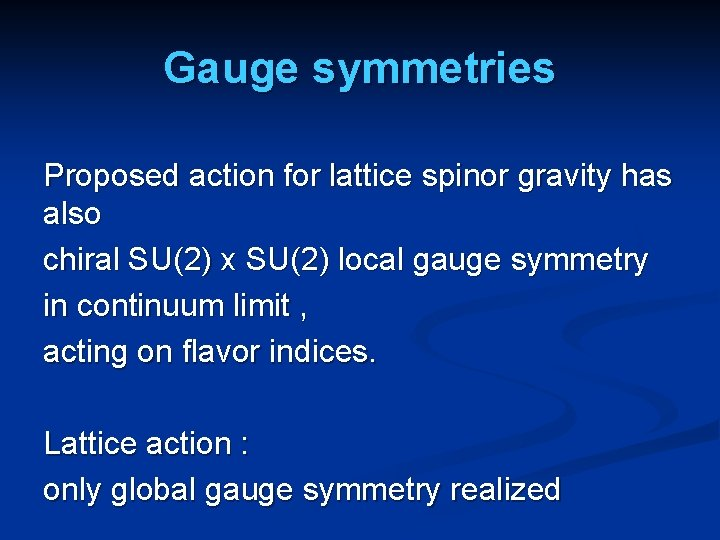Gauge symmetries Proposed action for lattice spinor gravity has also chiral SU(2) x SU(2) local gauge symmetry in continuum limit , acting on flavor indices. Lattice action : only global gauge symmetry realizedNext tasks Compute effective action for composite metric n Verify presence of Einstein-Hilbert term ( curvature scalar ) n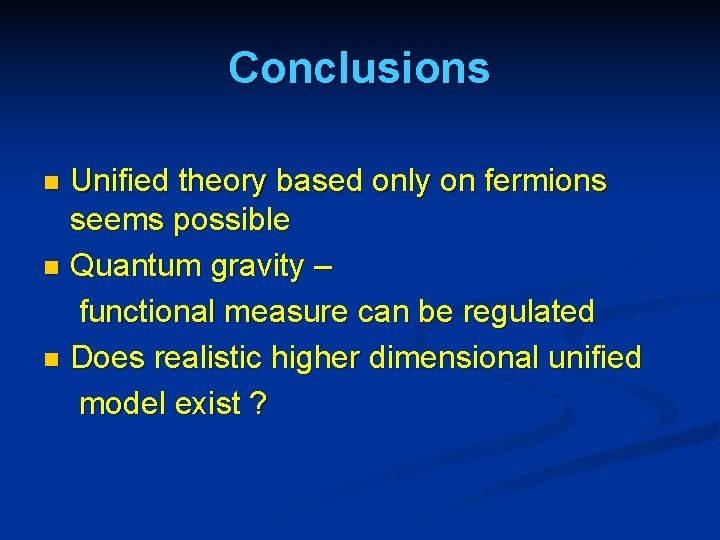Conclusions Unified theory based only on fermions seems possible n Quantum gravity – functional measure can be regulated n Does realistic higher dimensional unified model exist ? nend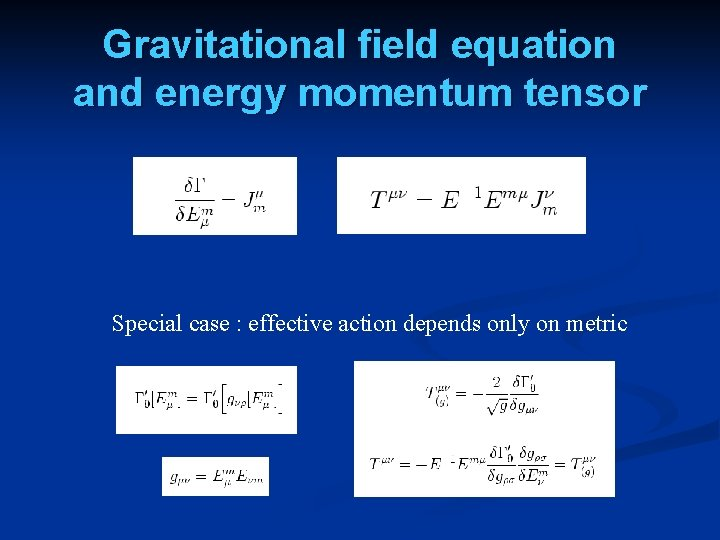Gravitational field equation and energy momentum tensor Special case : effective action depends only on metric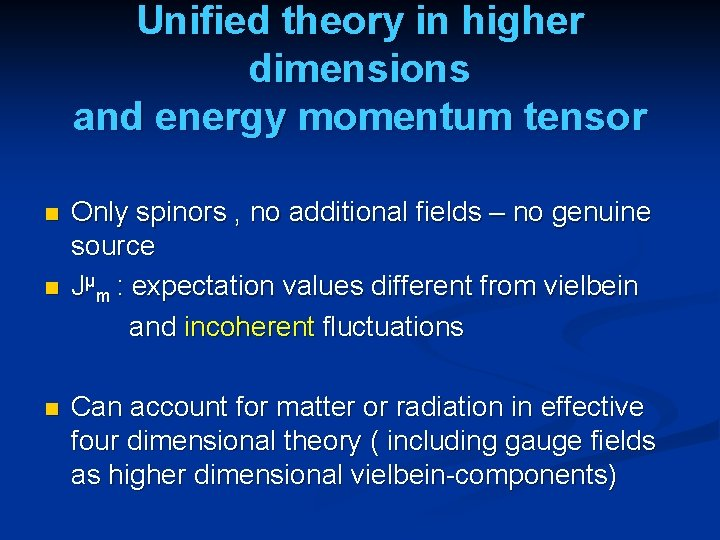Unified theory in higher dimensions and energy momentum tensor n n n Only spinors , no additional fields – no genuine source Jμm : expectation values different from vielbein and incoherent fluctuations Can account for matter or radiation in effective four dimensional theory ( including gauge fields as higher dimensional vielbein-components)